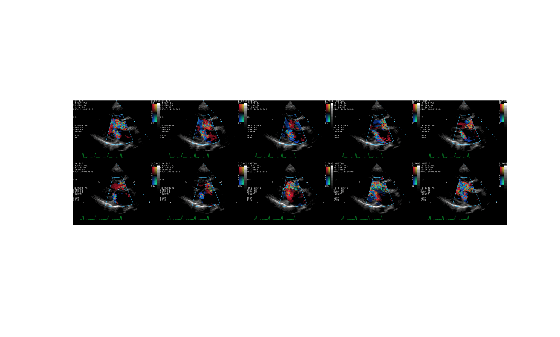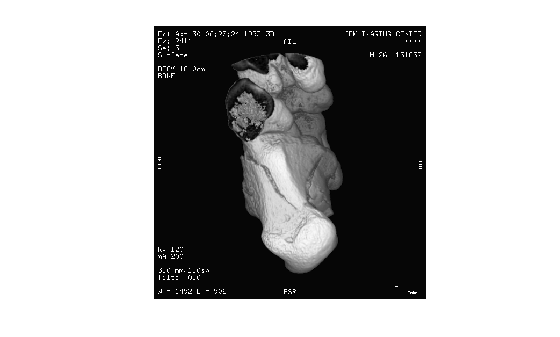## Syntax

``X = dicomread(filename)``
``X = dicomread(info)``
``X = dicomread(___,"frames",f)``
``X = dicomread(___,Name,Value)``
``[X,cmap] = dicomread(___)``
``[X,cmap,alpha] = dicomread(___)``
``[X,cmap,alpha,overlays] = dicomread(___)``

## Description

example

````X = dicomread(filename)` reads the image data from the compliant Digital Imaging and Communications in Medicine (DICOM) file `filename`. To read a group of DICOM files that contain a series of images that comprise a volume, use `dicomreadVolume`. ```
````X = dicomread(info)` reads DICOM image data from the message referenced in the DICOM metadata structure `info`.```
````X = dicomread(___,"frames",f)` reads only the specified frames `f` from the image, using any combination of input arguments from previous syntaxes.```
````X = dicomread(___,Name,Value)` reads DICOM image data using name-value arguments to configure the parser.```
````[X,cmap] = dicomread(___)` also returns the colormap, `cmap`.```
````[X,cmap,alpha] = dicomread(___)` also returns `alpha`, an alpha channel matrix for `X`.```
````[X,cmap,alpha,overlays] = dicomread(___)` also returns any overlays from the DICOM file.```

## Examples

collapse all

Read an indexed image from a DICOM file and display it using `montage`.

```[X,map] = dicomread("US-PAL-8-10x-echo.dcm"); montage(X,map,"Size",[2 5]);```Read an image from another DICOM file and display it using `imshow`.

```info = dicominfo("CT-MONO2-16-ankle.dcm"); Y = dicomread(info); figure imshow(Y,[]);```## Input Arguments

collapse all

Name of the DICOM file, specified as a character vector or string scalar.

Data Types: `char` | `string`

DICOM metadata, specified as a structure. You can create an `info` structure by using the `dicominfo` function.

Frames to read, specified as a positive integer scalar, a vector of positive integers, or `"all"`. When `f` is numeric, `dicomread` reads only the frames of the specified numbers from the image. By default, `dicomread` reads all frames of the DICOM image.

### Name-Value Arguments

Specify optional pairs of arguments as `Name1=Value1,...,NameN=ValueN`, where `Name` is the argument name and `Value` is the corresponding value. Name-value arguments must appear after other arguments, but the order of the pairs does not matter.

Example: `dicomread("CT-MONO2-16-ankle.dcm",UseVRHeuristic=false)` reads the image data from the DICOM file without using a heuristic.

Before R2021a, use commas to separate each name and value, and enclose `Name` in quotes.

Example: `dicomread("CT-MONO2-16-ankle.dcm","UseVRHeuristic",false)` reads the image data from the DICOM file without using a heuristic.

Read noncompliant DICOM files that switch value representation (VR) modes incorrectly, specified as a logical `1` (`true`) or `0` (`false`).

When set to `true`, `dicomread` uses a heuristic to help read certain noncompliant DICOM files that switch VR modes incorrectly. `dicomread` displays a warning if it uses this heuristic. If this heuristic is enabled, a small number of compliant files are not read correctly. Set `UseVRHeuristic` to `false` to read these compliant files.

Data Types: `logical`

## Output Arguments

collapse all

DICOM image, returned as one of these options:

• An m-by-n matrix representing a single-frame grayscale image, or an indexed image.

• An m-by-n-by-3 array representing a single-frame truecolor (RGB) image.

• A 4-D array representing a multiframe image.

Data Types: `int8` | `int16` | `uint8` | `uint16`

Colormap associated with image `X`, returned as one of these options:

• If `X` is an indexed image, then `cmap` is returned as a c-by-3 numeric matrix with values in the range [0, 1]. Each row is a three-element RGB triplet that specifies the red, green, and blue components of a single color of the colormap.

• If `X` is a grayscale or truecolor image, then `cmap` is empty (`[]`).

Data Types: `double`

Alpha channel matrix for image `X`, returned as an m-by-n matrix or 4-D array of nonnegative integers. The values of `alpha` are `0` if the pixel is opaque. Otherwise, they are row indices into `cmap`. To use `alpha`, you should substitute the RGB value in `cmap` for the value in `X`. The `alpha` output has the same height and width as `X`, and is 4-D for a multiframe image. `alpha` has the same data type as `X`.

Data Types: `int8` | `int16` | `uint8` | `uint16`

Overlays from the DICOM file, returned as an m-by-n matrix or 4-D array of binary values. Each overlay is a 1-bit black and white image with the same height and width as `X`. If multiple overlays are present in the file, then `overlays` is a 4-D multiframe image. If no overlays are in the file, then `overlays` is empty (`[]`).

Data Types: `logical`

## Tips

• This function reads imagery from files with any of these pixel formats:

• Little-endian, implicit VR, uncompressed

• Little-endian, explicit VR, uncompressed

• Big-endian, explicit VR, uncompressed

• JPEG (lossy or lossless)

• JPEG2000 (lossy or lossless)

• Run-length Encoding (RLE)

• GE implicit VR, LE with uncompressed BE pixels (1.2.840.113619.5.2)

## Version History

Introduced before R2006a